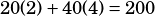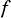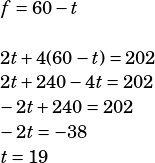SAT test 8 section question 10

Hoping you mean question 10 from the No Calculator section–that’s what I’m doing here. (If you meant 10 from the Calculator section, just submit another question.)

In this question, you can write two linear equations and solve, OR, you can backsolve! I’ll show backsolving first.

Start by trying answer choice C, which says there were 19 2-person tents. If there were 60 tents overall, that means there must have been 41 4-person tents, right?

Now, how many people would 19 2-person tents and 41 4-person tents fit?Whoa, we’re done in one try!

(Whenever I backsolve and the first answer I try is right, I feel like I should show you how I’d know an answer is wrong. If you try B, for example, you have 20 2-person tents and 40 4-person tents:, you don’t fit enough people so you need more of the bigger tents.)

Now, if you hate getting questions right the easy way, you can of course write equations. Sayis the number of 2-person tents andis the number of 4-person tents. You know there are 60 tents, so one of the equations isThe other equation has to do with the number of people those tents fit:Substitute and solve to find: